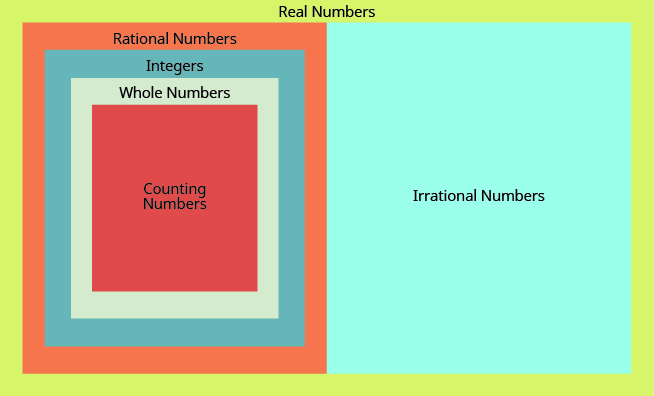Classifying Real Numbers

Learning Outcomes

• Classify real numbers as counting, whole, rational, irrational, or integers

We have seen that all counting numbers are whole numbers, all whole numbers are integers, and all integers are rational numbers. Irrational numbers are a separate category of their own. When we put together the rational numbers and the irrational numbers, we get the set of real numbers.

This diagram illustrates the relationships between the different types of real numbers.Real Numbers

Real numbers are numbers that are either rational or irrational.

Does the term “real numbers” seem strange to you? Are there any numbers that are not “real”, and, if so, what could they be? For centuries, the only numbers people knew about were what we now call the real numbers. Then mathematicians discovered the set of imaginary numbers. You won’t encounter imaginary numbers in this course, but you will later on in your studies of algebra.

example

Determine whether each of the numbers in the following list is a 1. whole number, 2. integer, 3. rational number, 4. irrational number, and 5. real number.

$-7,\Large\frac{14}{5}\normalsize ,8,\sqrt{5},5.9,-\sqrt{64}$

Solution:
1. The whole numbers are $0,1,2,3\dots$ The number $8$ is the only whole number given.

2. The integers are the whole numbers, their opposites, and $0$. From the given numbers, $-7$ and $8$ are integers. Also, notice that $64$ is the square of $8$ so $-\sqrt{64}=-8$. So the integers are $-7,8,-\sqrt{64}$.

3. Since all integers are rational, the numbers $-7,8,\text{and}-\sqrt{64}$ are also rational. Rational numbers also include fractions and decimals that terminate or repeat, so $\Large\frac{14}{5}\normalsize\text{and}5.9$ are rational.

4. The number $5$ is not a perfect square, so $\sqrt{5}$ is irrational.

5. All of the numbers listed are real.

We’ll summarize the results in a table.

Number Whole Integer Rational Irrational Real
$-7$ $\quad\checkmark$ $\quad\checkmark$ $\quad\checkmark$
$\Large\frac{14}{5}$ $\quad\checkmark$ $\quad\checkmark$
$8$ $\quad\checkmark$ $\quad\checkmark$ $\quad\checkmark$ $\quad\checkmark$
$\sqrt{5}$ $\quad\checkmark$ $\quad\checkmark$
$5.9$ $\quad\checkmark$ $\quad\checkmark$
$-\sqrt{64}$ $\quad\checkmark$ $\quad\checkmark$ $\quad\checkmark$

try it

The following mini-lesson provides more examples of how to classify real numbers.